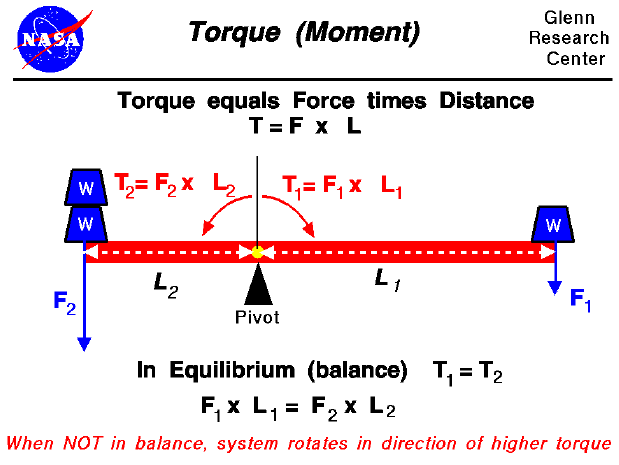A force may be thought of as a push or pull in a specific direction. When a force is applied to an object, the object accelerates in the direction of the force according to Newton's laws of motion. The object may also experience a rotation depending on how the object is confined and where the force is applied. A hanging door is an excellent example of this type of motion. When you push on a door it can not freely translate because it is confined (or pinned) by the hinges. It does, however, rotate on the hinges. The rotation itself depends on where you apply the force. As you get closer to the hinge, you must apply a larger force to make the door swing. As you get farther from the hinge, you can apply a smaller force to make the door swing.

The product of the force and the distance from a pivot (or hinge) is called the torque or the moment. Torques produce rotations in the same way that forces produce translations. Namely, an object at rest, or rotating at a constant angular velocity, will continue to do so until it is subject to an external torque. A torque produces an angular acceleration or change in angular velocity. If an object is not pinned, it rotates about its center of gravity when acted upon by an external force. The distance used in the calculation of the torque is then the distance from the center of gravity to the applied force.

As with forces, an object may be acted upon by multiple torques. The motion of the system then depends on the net torque on the system. In the figure we have a beam on which some weights are sitting. The beam itself sits on a pivot. To the right a single weight (W) produces a force (F1) acting at a distance (L1) from the pivot. This creates a torque (T1) equal to the product of the force and the distance.

T1 = F1 * L1

To the left of the pivot two weights (W) produce a force (F2) at a distance (L2). This produces a torque (T2) in a direction opposite from T1 because the distance is in the opposite direction.

T2 = F2 * L2

If the system were in equilibrium, or balanced, the torques would be equal and no net torque would act on the system.

T1 = T2 or T1 - T2 = 0

F1 * L1 = F2 * L2

If F2 = 2 * F1, what is the relation between L1 and L2 to balance the system? If F2 = 2 * F1, and L1 = L2, in which direction would the system rotate?

Aeronautical engineers use the torque generated by aerodynamic surfaces to stabilize and control aircraft. On airplanes, the control surfaces produce aerodynamic forces. These forces are applied at some distance from the aircraft cg and therefore cause the aircraft to rotate. The elevators produce a pitching moment, the rudder produce a yawing moment, and the ailerons produce a rolling moment. The ability to vary the amount of the force and the moment allows the pilot to maneuver or to trim the aircraft. On model rockets, the fins are used to generate a torque about the rocket center of gravity to provide stability during powered flight. On kites, the aerodynamic and weight forces produce a torque about the bridle point. The distance from the bridle point and the magnitude of the forces has a strong effect on the performance of the kite.Guided Tours